Q1.  What does an electric circuit mean?
Ans. A continuous conducting path consisting of wires and other resistances and a switch between the two terminals of a cell or a battery along which an electric current flow is called a circuit.
Q2.  Define the unit of current.
Ans. The unit of current is Ampere. 1 Ampere is said to be when 1 coulomb of charge flow through any cross section of a conductor in 1 second.
Q3.  Calculate the number of electrons constituting one coulomb of charge.
Ans. 1C = 6 × 1018 electrons.
Q4.  Name a device that helps to maintain a potential difference across a conductor.
Ans. A battery of many cells or a single cell can maintain potential difference.
Q5.  What is meant by saying that the potential difference between two points is 1 V?
Ans. The potential difference between two points is said to be 1 V if 1 Joule of work is done in moving 1 Coulomb of charge from one point to another point.
Q6.  How much energy is given to each coulomb of charge passing through a 6 V battery?
Ans. Amount of charge = 1 coulomb or 1C
Potential difference = 6V
Energy or work done = ?
Work done or energy = V × Q[Q is the amount of charge flowing between two points at potential difference V = 6]
i.e., W = V × Q
W = 6 × 1
Work done or energy = 6 joules.
Q7.  On what factors do the resistance of a conductor depend?
Ans. Resistance of a conductor depends on:
(a)  Length of a conductor. Resistance of a conductor is directly proportional to the length of a conductor. If length increases resistance will also increase.
(b)  Area of cross section of a conductor. The resistance of a conductor is inversely proportional to the area of the cross section of a conductor.
(c)  Effect of material of a conductor. The resistance of a conductor also depends on the material of a conductor.
E.g. The resistance of nichrome wire is 60 times more than that of copper wire as nichrome has high electrical resistance.
(d)  Effect of temperature. The resistance of all pure metals increases on increasing the temperature and decreases on decreasing the temperature.
Q8.  Will current flow more easily through a thick wire or a thin wire of the same material, when connected to the same source? Why?
Ans. The current will flow more easily through a thick wire as compared to the thin wire because the resistance of thick wire is less than that of thin wire. Less resistance, means more current.
Q9.  Let the resistance of an electrical component remains constant while the potential difference across the two ends of the component decreases to half of Us fanner value. What change will occur in the current through it?
Ans. Amount of current will be halved.
Q10.  Why are coils of electric toasters and electric irons made of an alloy rather than a pure metal?
Ans. The coils of such heating appliances are made up of an alloy rather than a pure metal because:
(a)  The resistivity of an alloy is much higher than that of a pure metal.
(b)  An alloy does not undergo oxidation easily even at high temperature when it is even red hot.
Q11.  (a) Which among iron and mercury is a better conductor?
(b)  Which material is the best conductor?
Ans. (a)  Iron
(b)  Silver
Q12.  Draw a schemata: diagram of a circuit consisting of a battery of three cells of 2 V each, a 5 Ω LI resistor, 8 Ω resistor, and a 12 Ω resistor, and a plug key, alt connected in seriess.
Ans.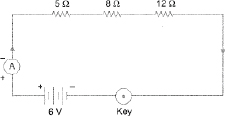Q13.  Redraw the circuit of Question 12, putting in an ammeter to measure the current through the resistors and a voltmeter to measure the potential different across the 12 Ω resistors. What would be the readings in the ammeter and the voltmeter?Rs = 5 Ω + 8 Ω + 12 Ω = 25 Ω
V = 6 V
I = ?= 0.24 A
When R = 12 Ω
V = IR
= 0.24 x 12
= 2.88 V
Hence, reading in the ammeter would be 0.24 A and reading in voltmeter would be 2.88 V
Q14.  Judge the equivalent resistance when Die following are connected in parallel—
(a) 1 Ω , 106 Ω , (b) 1 Ω , 103 Ω and 106 Ω.
Ans. (a)  1 ohm and 106 times ohm
R1 = 1 ohm
R2 = 106 times = 1000000 ohm
Total resistance in parallel 1/R = 1/R1 + 1/R2
= 1/1 + 1/1000000
= 1000000+1/1000000
= 1000001/1000000
= 1/R = 1/1 ohm (approx)
Resistance = 1 ohm (approx)
(b)  R1 = 1 ohm
R2 = 103 ohm
R3 = 106 ohm
Total resistance in parallel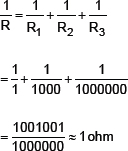Q15.  An electric lamp of 100 Ω, a toaster of resistance. 50 Ω , and a water filter of resistance 500 Ω are connected in parallel to a 220 V source. What is the resistance of an electric iron connected to the same source that takes as much current as all the three appliances, and what is the current through it?
Ans. Resistance of electric lamp =100 ohm
Resistance of a toaster = 50 ohm
Resistance of a water filter = 500 ohm
Potential difference = 220 volt
Total resistance of the three appliances in parallel = 1/R = 1/R1 + 1/R2 + 1/R3
= 1/100 + 1/50 + 1/500
1/R = 5 + 10 + 1/500
1/R = 16/500 = 4/125
= 125/4 = 31.25 ohm
V = I × R
220 = I × 31.25
I = 7.04 amp
Hence current through the electric iron = 7.04 amp.
Q16.  What are the advantages of connecting electrical devices in parallel with the battery instead of connecting them in series?
Ans. Advantages of parallel connection are:
(a)  In parallel circuit, if one electric appliance stop working due to some defect, then all other appliances keep working normally.
(b)  In parallel circuit, each electrical appliance has its own switch due to which they can be turned on or off without affecting other appliances.
(c)  Each electrical appliance get the same voltage (220 V) as that of the power supply line.
(d)  In parallel connection of electrical appliances, the overall resistance of the circuit is reduced due to which the current from the power supply is high.
Q17.  How can three resistors of resistances 2 Ω , 3 Ω , and 6 Ω be connected to give a total resistance of (a) 4 Ω , (b) 1 Ω?
Ans. R1 = 2 ohm
R2 = 3 ohm
R3 = 6 ohm
(a)  When R2 and R3 are connected in parallel with R1 in series we get
1/R = 1/R2 + 1/R3
g= 1/3 + 1/6
= 1/2
Thus, R = 2 ohm
Resistance in series = R + R1
= 2 + 2
= 4 ohm
(b)  When R1,R2, R3 are connected in parallel we get
1/R = 1/R1 + 1/R2 + 1/R3
= 1/2 + 1/3 + 1/6
= 1 ohm.
Q18.  What is (a) the highest, (b) the lowest total resistance that can be secured by combinations of four coils of resistance 4 Ω , 8 Ω , 12 Ω , 24 Ω?
Ans. (a) The highest resistance is when the resistances are connected in series:
R1 = 4 ohm                R2 = 8 ohm
R3 = 12 ohm                R4 = 24 ohm
Total resistance in series = R1 + R2 + R3 + R4
= 4 + 8 + 12 + 24
= 48 ohm
Thus, highest resistance is 48 ohm.
(b)  The lowest resistance is when the resistances are connected in parallel
Total resistance in parallel
= 1/R1 + 1/R2 + 1/R3 + 1/R4
1/R = 1/2 + 1/8 + 1/12 + 1/24
= 12/24
1/R = 1/2 ohm, R = 2 ohm
Thus, lowest resistance is 2 ohm.
Q19.  Why does the cord of an electric heater no, glow while the heating element does?
Ans. The resistance of cord is extremely small as compared to that of heating element, so the heat produced in cord is less as compared to heating element. So the heating element begins to glow but cord does not glow.
Q20.  Compute the heat generated while transferring 96000 coulomb of charge in one hour through a potential difference of 50 V.
Ans. Charge = 96000 coulomb
Time = 1 hour = 3600 seconds
Potential difference = 50 V
I = Q/T
I = 96000/3600 = 80/3 amp
V = I × R
50 = 80/3 × R
Thus. R = 15/8 ohm
Heat = I2RT
= (80/3)2 × 15/8 × 3600
= 800 × 6000
= 4800000 joules.
Q21.  An electric iron a, resistance 20 Ω takes a current of 5 A Calculate the heat developed in 30 s.
Ans. Resistance of the iron = 20 ohm
Current = 5 amp
Time = 30 seconds
Heat produced = I2RT
= 52 × 20 × 30
= 15000 joules
Q22.  What determines the rate at which energy is delivered by a current?
Ans. Electrical power determines the rate at which the energy is delivered by a current.
Q23.  An electric motor takes 5 A from a 220 V line. Determine the power of the motor and the energy consumed in 2h.
Ans. Current in the motor, I = 5 amp
Potential difference, V = 220 V
Time = 2 hours
Power of the motor = V × I
= 220 × 5
1100 watt or 1.1. kWh
Energy = power × time
= 1.1 kWh × 2 h.
= 2.2 kWh
QUESTIONS FROM NCERT TEXTBOOK
Q1.  A piece of wire of resistance R is cut into five equal parts. ‘These parts are then connected in parallel. If the equivalent resistance of this combination is R’, then what is the ratio R/R’?
Ans. 25 : 1
Q2.  Which of the following terms does not represent electrical power in a circuit?
(a)  I2R
(b)  IR2
(c)  W
(d)  V2/R
Ans. (b) IR2
Q3.  An electric bulb is rated 220 V and 100 W. When it is operated on 110 V, power consumed will be—
(a)  100 W
(b)  75 W
(c)  50 W
(d)  25 W
Ans. (d) 25 W.
Q4.  Two conducting wires of the same material and of equal lengths and equal diameters are first connected in series and then parallel in a circuit across the same potential difference. The ratio of heat produced in series and parallel combinations would be—
(a)  1 : 2
(b)  2 : 1
(c)  1 : 4
(d)  4 : 1
Ans. (c) 1 : 4
Q5.  How is a voltmeter connected in the circuit to measure the potential difference between two points?
Ans. It is connected in parallel.
Q6.  A copper wire, has diameter 0.5 mm and resistivity of 1.6 × 10V8 Ω m. What will be the length of this wire to make its resistance 10 Ω ? How much does the resistance change if the diameter is doubled?
Ans. Diameter = 0.5 mm = 0.0005 m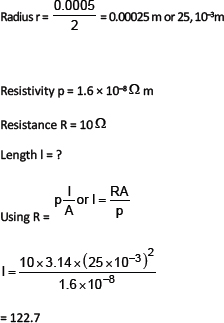The resistance becomes one-fourth if the diameter is doubled.
Q7.  The values of current I flowing in a circuit with resistor for the corresponding values of potential difference V across the resistors are given below:
I (amperes) 0.5   1.0   2.0   3.0   4.0
V(volts) 1.6   3.4   6.7   10.2   13.2
Plot a graph between V and I and calculate the resistance of that resistor.
Ans.Resistance of resistor R (mean)Q8.  When a 12 V battery is connected across an unknown resistor, there is a current 2.5 inA in the circuit. Find the value of the resistance of the resistor.
Ans. V = 12 V
I = 2.5 mA = 2.5 x 10-3 A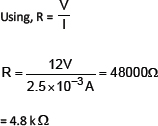Q9.  A battery of 9 V is connected in series with resistors of 0.2 Ω , 0.3 Ω , 0.4 Ω , 0.5 Ω and 12 Ω respectively. How much current would flow through the 12 Ω resistor?
Ans. V = 9 Y
Rs = 0.2 Ω + 0.3 Ω . + 0.4 Ω + 0.5 Ω + 12 Ω.
= 13.4 Ω
I = ?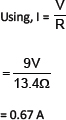Q10.  How many 176 Ω resistors (in parallel) are required to carry 5 A on a 220 V line?
Ans. I = 5 A V = 220 V
Let the number of 176 Ω resistors be n.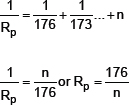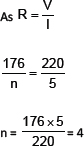Hence, four resistors of 176 W are required to carry 5 A on a 220 V line.
Q11.  show how you would connect three resistors each of resistance 6 Ω , so that the combination has a resistance of (i) 9 Ω , (U) 4 Ω.
Ans. (i) To get 9 Ω �XConnect 2 resistors of 6 Ω in parallel and third resistor in series.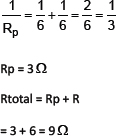(ii)  To get 4 Ω —Connect 2 resistors in series and the third in parallel to both of them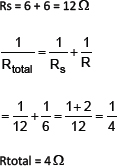Q12.  Several electric bulbs designed to be used on a 220 V electric supply line, are rata 10 W. How many lamps can be connected in parallel to each other across (he two win. of a 220 V line if the maximum allowable current is 5 A?
Ans. P = 10 W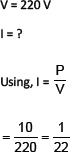If number of bulbs connected to current of 5 A is n. Then,n = 5 � 22 = 110
110 bulbs can be attached.
Q13.  A hot plate of an electric oven connected to a 220 V line has two resistance coils A and B, each of 24 Ω resistance, which may be used separately, in series, or in parallel. What are the currents in the three cases?
Ans. (i) When used separately(ii)  When connected in series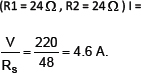(iii)  When connected in parallel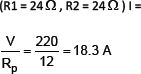Q14.  Compare the power used in the 2 Ω resistor in each of the following circuits:
(i)  a 6 V battery in series with 1 Ω and 2 Ω resistors, and
(ii)  a 4 V battery in parallel with 12 Ω and 2 Ω resistors.
Ans. (i) V = 6 V
R1 = 1 Ω series with R3 = 2 Ω . P = ?
R2 = 2 Ω
R = R1 + R2 + R3
=1 + 2 = 30Comparison: Power used by 2 Ω resistor in both the circuits are same, i.e. 8 W.
Q15.  Two lamps, one rated 100 W at 220 V, and the other rated 60 W at 220 V, are connected in parallel to electric mains supply. What current is drawn from the line if the supply voltage is 220 V?
Ans. P1 = 100 w
P2 = 60 W
P = P1 + P2 = 160 W
V = 220 V
I = ?Q16.  Which uses more energy, a 250 W TV set in 1 hr, or a 1200 W toaster in 10 minutes?
Ans. 250 W TV set in an hour
Q17.  An electric heater of resistance 8 Ω draws 15 A from the service mains for 2 hours. Calculate the rate of which heat is developed in. the heater
Ans. 1800 Wall.
Q18.  Explain the following:
(a)  Why the tungsten a used almost exclusively for the filament of electric lamps?
Ans. •  It has a special property that it glows on heating.
•  It has ,i high melting point.
•  It does not tenet with the gases present in air and does not get oxidized.
•  It has a low resistance.
(b)  Why are the conductors of electric heating devices, such as bread-toasters and electric irons, made up of alloys rather than a pure menu?
Ans. •  They offer higher resistance than pure metals, thus heating better.
•  They have a high melting point.
•  They do not oxidize.
(c)  Why is the series arrangement not used for domestic circuits?
Ans. •  If any appliance is at fault, then the current is not able to flow through.
•  Different appliances have different current requirements, but in series the current is the same. This can cause a power surge, thus damaging the appliances.
•  Very long wires m domestic series circuits offer unusually high resistance, which is unwanted.
(d)  How does the resistance of a wire vary with its area of cross section?
Ans. The relation between the resistance of a wire and its area of cross-section can be defined as:
•  R is inversely proportional to the area of cross-section(e)  Why copper and aluminium wires are usually employed for electricity transmission?
Ans. •  Copper and aluminium are much more easily and cheaply available than other metals.
•  They offer low resistance and do not get heated.
•  They can be easily made into wires due to high malleability.